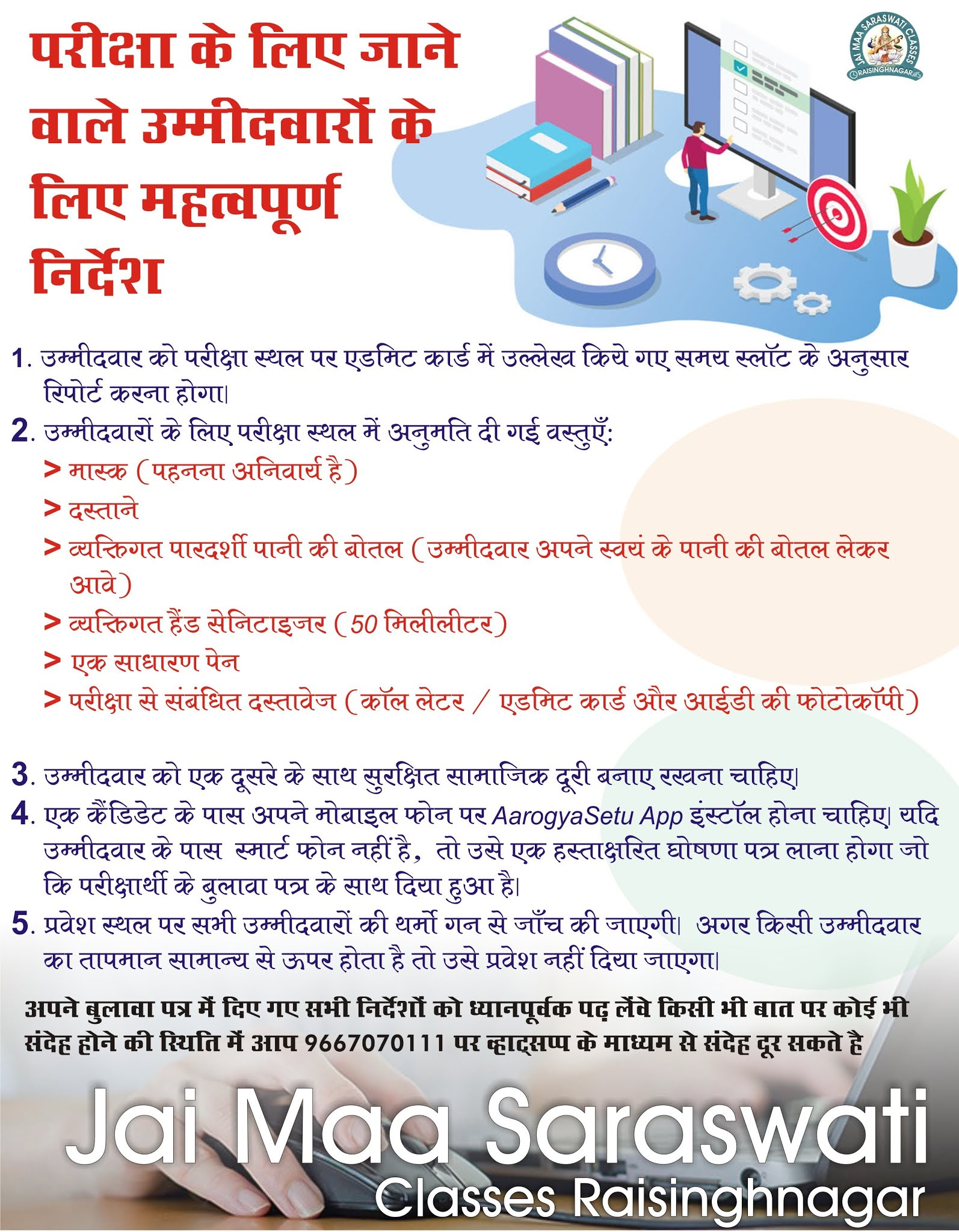### English Quiz

Here is a quiz on English Language being provided by JMS to let you practice the best of latest pattern English Questions for upcoming Banking Exams.

Directions (1-15): In the following questions two columns are given containing three sentences/phrases each. In first column, sentences/phrases are A, B and C and in the second column the sentences/phrases are D, E and F. A sentence/phrase from the first column may or may not connect with another sentence/phrase from the second column to make a grammatically and contextually correct sentence. Each question has five options, four of which display the sequence(s) in which the sentences/phrases can be joined to form a grammatically and contextually correct sentence. If none of the options given forms a correct sentence after combination or none of them connects, mark (e), i.e. “None of the above” as your answer.

Q1.

A-E
A-E and C-D
B-F and C-D
B-F
None of the above
Solution:
'B-F' and 'C-D' are the correct combinations here and thus make meaningful sentences.

Q2.

B-D
A-F
A-F, C-E and B-D
A-F and C-E
None of the above
Solution:
'A-F' and 'C-E' are the correct combinations here and thus make meaningful sentences.

Q3.

A-E, B-F and C-D
A-E and C-D
A-E and B-F
C-D
None of the above
Solution:
'A-E' and 'B-F' are the correct combinations here and thus make meaningful sentences.

Q4.

A-E
B-F
C-D
A-E and B-F
None of the above
Solution:
'C-D' is the correct combination here and thus makes a meaningful sentence.

Q5.

A-E, C-F and B-D
B-F
C-D and B-F
C-D, B-F and A-E
None of the above
Solution:
'A-E', 'B-F' and 'C-D' are the correct combinations here and thus make meaningful sentences.

Q6.

C-D
A-E
A-E and B-F
B-F
None of the above
Solution:
'C-D' and 'A-E' are the correct combinations here and thus make meaningful sentences.

Q7.

A-E and B-F
A-E, C-D and B-F
B-F and C-D
C-E, A-F and B-D
None of the above
Solution:
'A-E', 'B-F' and 'C-D' are the correct combinations here and thus make meaningful sentences.

Q8.

A-F, B-D and C-E
B-D and C-E
B-D
A-F and C-E
None of the above
Solution:
'A-F' and 'C-E' are the correct combinations here and thus make meaningful sentences.

Q9.

A-E and B-F
A-E
C-D
C-D and B-F
None of the above
Solution:
None of the above

Q10.

C-E
A-F, B-D and C-E
A-F and B-D
B-D
None of the above
Solution:
'A-F', 'C-E' and 'B-D' are the correct combinations here and thus make meaningful sentences.

Q11.

C-D and A-E
A-E and B-F
C-D
B-F
None of the above
Solution:
'C-D' is the correct combination here and thus makes a meaningful sentence.

Q12.

B-F
A-E
C-D
A-E and B-F
None of the above
Solution:
None of the above

Q13.

A-E and B-F
C-D
C-D and B-F
B-F
None of the above
Solution:
'B-F' and 'C-D' are the correct combinations here and thus make meaningful sentences.

Q14.

A-E and B-F
A-E, B-F and C-D
C-D
B-F
None of the above
Solution:
'A-E', 'B-F' and 'C-D' are the correct combinations here and thus make meaningful sentences.

Q15.

A-E
A-E and C-D
C-D
B-F
None of the above
Solution:
None of the above

Ⓒ 2019. JMS Classes Raisinghnagar
✆ 9667070111

Designed By : Satnam Gill